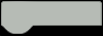# Project 4 Breathing LEDsTill now, we have learned how to control an LED connected onto Arduino by programming. In fact, we can do way too much with Arduino. In this project we are going to control the brightness of LEDs on Arduino. There are 6 digital pins marked with “~” on the UNO board: 3, 5, 6, and 9. This mark indicates that these pins can be used to generate PWM. When the circuit slowly fades an LED on and off, it forms an effect of breathing, so we call it breathing LEDs.The connection is the same as the one of project 2.Connect the board with your computer, open Mind+ and load the Arduino library. Input the example program below:

Example Program 4-1:Load the codes to your Arduino board then you will see the LED gradually brightening and dimming.CODE
``````1.volatile float mind_n_value;
2.// function declaration
5.
6.
7.// Main program starts
8.void setup() {
9.
10.}
11.void loop() {
14.}
15.
16.
17.// define function
19.    mind_n_value = 255;
20.    while (!(mind_n_value<=0)) {
21.        analogWrite(10, mind_n_value);
22.        delay(20);
23.        mind_n_value -= 5;
24.    }
25.}
27.    mind_n_value = 0;
28.    while (!(mind_n_value>=255)) {
29.        analogWrite(10, mind_n_value);
30.        delay(20);
31.        mind_n_value += 5;
32.    }
33.}  ``````

We have been familiar with most codes such as, variable declaration, pin setup,and function call.

There are two functions fadeOff and fadeOn in main function, and they are basically all the same except the parameters.

CODE
``````19.void fadeOff() {
20.    mind_n_value = 255;
21.    while (!(mind_n_value<0)) {
22.        analogWrite(10, mind_n_value);
23.        delay(20);
24.        mind_n_value -= 5;
25.    }
26.}``````

There is a new statement in fadeOff function: while. The while loop is used to repeat a section of code an unknown number of times until a specific condition is met.The while statement includes another new function:

￭ analogWrite( 10 ,mind_n_value );

How to send analog values to a digital pin? This function is the answer. The premise to use the function is to get a digital pin with PWM function. The 6 pins (3, 5, 6, 9, 10, 11) can be used to output PWM signal.PWM

PWM is a technique for getting analog results with digital means. Digital control is used to create a square wave, a signal switched between on and off. This on-off pattern can simulate voltages in between full on (5V) and off (0V) by changing the portion of the time the signal spends on versus the time that the signal spends off. The duration of “on time” is called the pulse width.

We can learn it well through the graphical below.In the graphical above, the green lines represent a regular time period. This duration or period is the inverse of the PWM frequency. In other words, with Arduino’s PWM frequency at about 500Hz, the green lines would measure 2 milliseconds each. A call to analogWrite() is on a scale of 0-255, such that analogWrite(255) requests a 100% duty cycle (always on), and analogWrite(127) is a 50% duty cycle (on half the time) for example.

PWM can be used to adjust the brightness of LED or the speed of motor, for instance, controlling an Arduino-based robot car.1. Type the codes of this chapter into Mind+, compile and debug.2. Use LEDs to create an effect of flickering flame by controlling its brightness via PWM.

Main components:

Red LED ×1

Yellow LED ×2

220Ω Resistor ×1

The function random() can be very useful in this project. It can generate a random within a designated range.

Note: set a brightness value for the LED first, and then generate a random around it. For example, random(120)+135. The related block should be:The brightness of the LED will be changed constantly around 135.